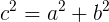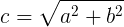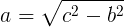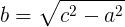# Pythagorean theorem free online calculator

Hypotenuse (c) calculation:

Leg (a) calculation:

Leg (b) calculation:

## Pythagorean theorem

For right triangle: the square value the hypotenuse (c) is equal to the sum of the square value of leg (a) and the square value of leg (b):### Hypotenuse (c) calculation### Leg (a) calculation### Leg (b) calculationCurrently, we have around 5611 calculators, conversion tables and usefull online tools and software features for students, teaching and teachers, designers and simply for everyone.